Want to share your content on R-bloggers? click here if you have a blog, or here if you don't.sine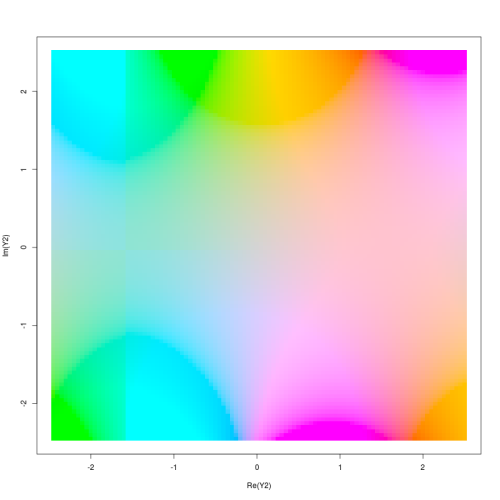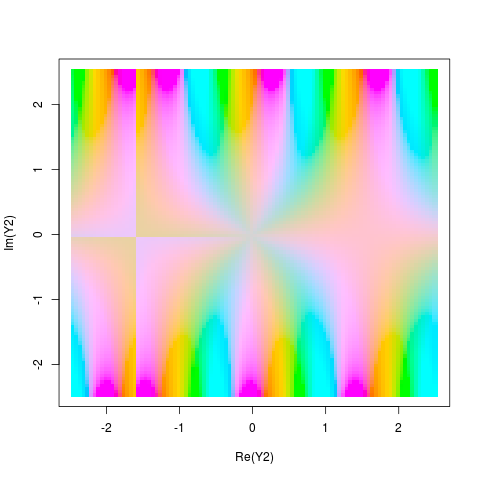mis-calculated the angles on sinemid-res, small cex, pch=46, sine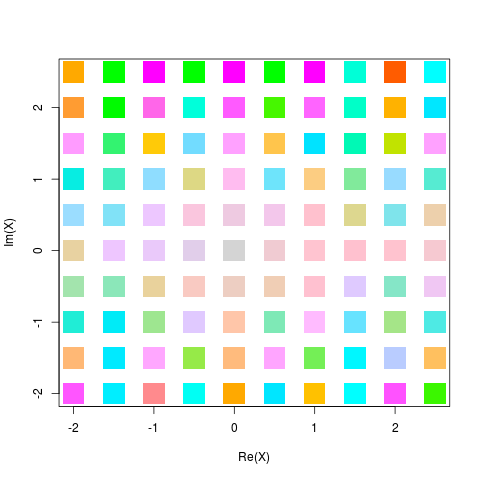low-res, small cex, pch=46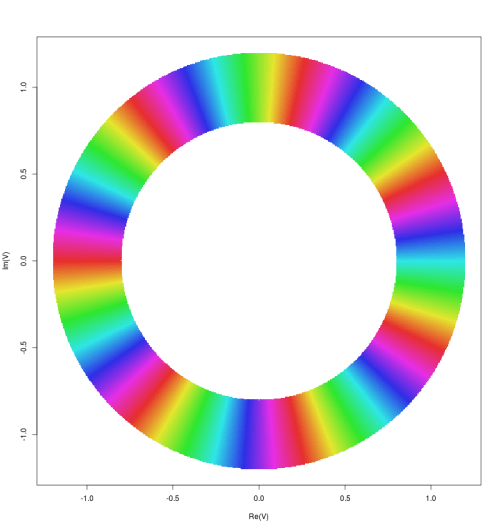z^17th powersine in HCL rather than HSV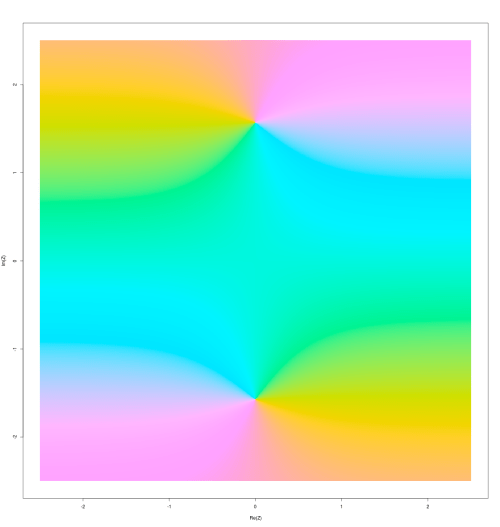cosh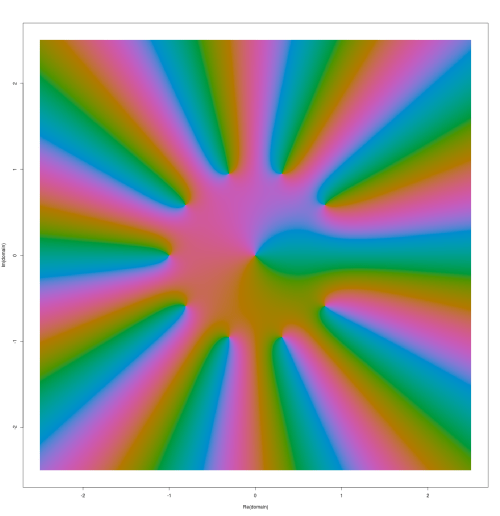z+zz+zzz+zzzz+zzzzz+zzzzzz+zzzzzzz+zzzzzzzz+zzzzzzzzz+zzzzzzzzzz

playing along with Elias Wegert in R:

```X <- matrix(1:100,100,100)                  #grid
X <- X * complex(imaginary=.05) + t(X)/20    #twist & shout
X <- X - complex(real=2.5,imaginary=2.5)     #recentre
plot(X, col=hcl(h=55*Arg(sin(X)), c=Mod(sin(X))*40 ) ,        pch=46, cex=6)
```

Found it was useful to define these few functions:

```arg <- function(z) (Arg(z)+pi)/2/pi*360     #for HCL colour input
ring <- function(C) C[.8 < Mod(C) &   Mod(C) < 1.2]        #focus on the unit circle
lev <- function(x) ceiling(log(x)) - log(x)
m <- function(z) lev(Mod(z))
plat <- function(domain, FUN) plot( domain, col= hcl( h=arg(FUN(domain)), l=70+m(domain)), pch=46, cex=1.5, main=substitute(FUN) )           #say it directly
```

NB, `hcl`‘s hue`[0,360]` so `phase` or `arg` needs to be matched to that.